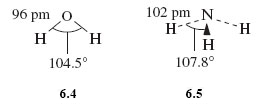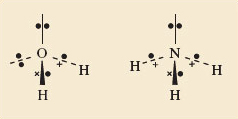Science, Maths & Technology

### Become an OU studentDiscovering chemistry

Start this free course now. Just create an account and sign in. Enrol and complete the course for a free statement of participation or digital badge if available.

# 3.3 The geometry of the water and ammonia molecules

To start with apply the logic introduced in section 3.2, and think about possible structures for H2O and NH3.

• What would be the shapes of the H2O and NH3 molecules if they were dictated only by bond-bond repulsions?

• The two O—H bonds of H2O and the three N—H bonds of NH3 would get as far apart as possible: H2O would be linear like BeF2, and NH3 would be trigonal planar like BF3 (see Figure 7).

The observed (experimentally determined) shapes are shown in Structures 6.4 and 6.5.

H2O is V-shaped and NH3 is pyramidal. In both cases the inter-bond angle is much closer to the tetrahedral angle of 109.5° than to the possible value of 180° and 120°, respectively.• What is present in H2O and NH3 that might explain these differences?

• In H2O and NH3, the O and N, carry non-bonded electron pairs.

You have previously looked at the Lewis structures for these molecules, and noted that in order to make up an octet of electrons around the central atoms you had ‘left over’ non-bonded electrons.

Specifically, for H2O around the oxygen atom there are two bonding pairs of electrons and two non-bonded pairs. And in NH3 there are three bonding pairs and one non-bonded pair.

Thus, both central atoms are surrounded by four pairs of electrons, and if these four pairs repel one another, they will be directed towards the corners of a tetrahedron like the four C—F bonds of CF4 in Figure 7c. The resulting arrangements are shown in Figure 9, both consistent with the idea that the four pairs of electrons around the central atoms try to get as far apart as possible. The non-bonded pairs as well as the bonding pairs are involved in this repulsionFigure 9 The shapes of the H2O (left) and NH3 (right) molecules

Overall the prediction is for a V-shaped H2O and a pyramidal NH3 molecule, with inter-bond angles close to the tetrahedral angle.